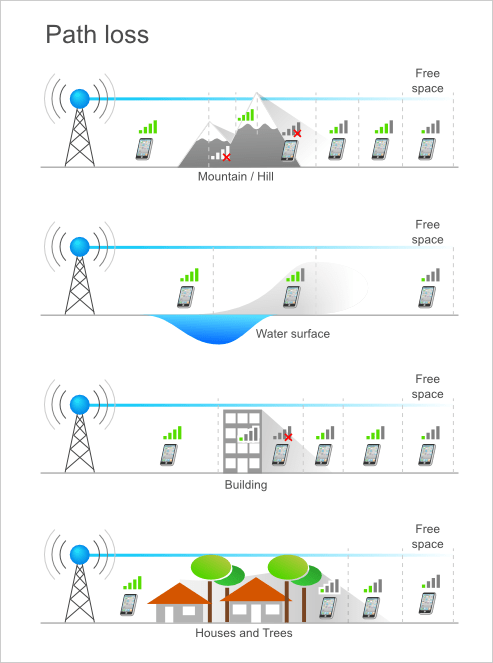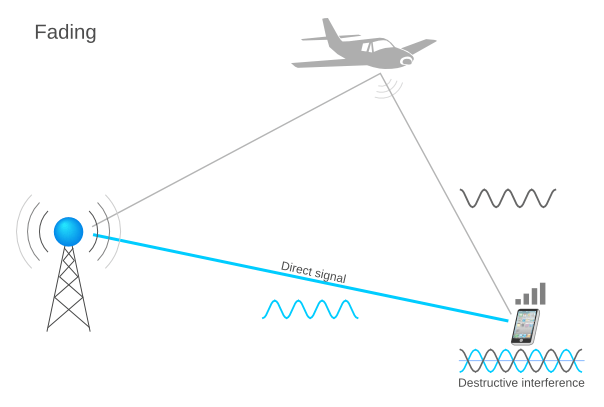Contents

## Power

One of the radio propagation concepts it the concept of power. In physics, it refers to the an amount of energy consumed per unit time. There are various units of measuring power: the joule per second, the watt, the horsepower etc.

In radio, microwave and fiber optic networks the unit of measure of power is the Decibel-milliwatt (dBm). The dBm is an electrical power unit in decibels (dB) referenced to 1 milliwatt (mW).

Here is the formula for representing the power in dBm:

P(dBm) = 10 · log10(P(mW) / 1mW)

The transmit power (Tx) is the energy transmitted through a specific bandwidth, generated by the radio into the Radio Frequency (RF). Tx power is typically measured in dBm or W.

The receive power (Rx) is the energy of the receive signal and is also measured in dBm.

Below you have a conversion table with a few examples.

dBmLinear
0 dBm1 mW
30 dBm1 W
-30 dBm1 μW

## Path Loss

Path loss, as a radio propagation concept, refers to the phenomenon of power density decrease of an electromagnetic wave, as it propagates through space. Path loss is a determining factor in analyzing the link budget (accounting of all gains and losses from the transmitter to the receiver through a particular medium)of a telecom system.

Path loss can be caused by various factors: refraction, diffraction, free-space loss, reflection, aperture-medium coupling loss or absorption. Other variables in determining the path loss are the environment, the type of terrain, the medium of propagation, the distance between the emitter and the receiver or the type and mounting of the antennas.

Path losses occur during the natural expansion of radio waves in the free space, when the signal is obstructed by an impenetrable obstacle or when the signal’s medium of transmission is not transparent to electromagnetic waves.

Multipath is an effect related to path loss and is caused by a signal transported from the transmitter to the receiver through multiple different paths. Thus, the signal arriving at the receiver is variable, depending on the distribution of intensity, and the propagation time, and the bandwidth of the transmitted signal.

Small scale fading is another phenomenon caused by rapid changes in the radio signal amplitude in a short time frame or on a short distance.

### Prediction Models

Free space path loss, also expressed as 1/r2 is an elementary model to be considered when designing a radio communications system. It is the standard free space loss caused by the expanding wavefront area, as the wave travels through free space.

Other widely used path loss prediction models are: Hata, Cost231 or Walfisch-Ikegami. They are based on measured and averaged losses through various classes of radio links.Link margin is the result of the difference between the receiver’s sensitivity and the the actual received power, and is measured in dB.

It is a system performance parameter.

The link margin value indicates three distinct outcomes:

• link margin equals 0 – the system is on the edge of proper functioning
• link margin is greater than 0 – the system can tolerate a certain amount of attenuation of the RF power
• link margin is less than 0 – the system is not set up properly and cannot transfer the information; a receiver with better sensitivity is needed

Link budget takes into account all the gains and losses from the transmitter to the receiver from: path losses, antennas, antenna feeders, power levels and receiver sensitivity. It is a key element in designing a mobile network and ultimately leads to a network design that functions correctly according to all requirements at a reasonable cost.

Link budget can be calculated with the below formula:

RP = TP + G − L

where: RP – received power (dBm)

TP – transmitted power (dBm)

G – gains (dB)

L – losses (dB)

The factors that determine link budget can vary in time, as path loss does, and, in such cases, the worst case scenario will be taken into account. The link budget calculation essentially leads to determining the cell size by accounting for the maximum allowed path loss without affecting the communication quality.

In mobile telecommunications, the range is the usable distance determining the reach of the radio wave propagation.To find out what the range is in a mathematical manner, the equation below may be used:

PR = PT + G − LP

PT – transmitted power

G – the combined antenna gains at Tx and Rx

LP – path loss.

The range is defined as the maximum distance at which the received power (PR) is greater than the sensitivity, which can be symbolized as PS , in both uplink and downlink. Path loss (LP) increases with distance, and is symmetric in uplink and downlink, but since the transmitted power (PT) and the received power (PR) are different, the link itself may not be symmetric. Therefore, the range of a basestation is determined as the distance that allows a maximum path loss value without losing connectivity.

The formula used above is also a simplified version of calculating link budget.

The range is variable and various factors influence it:

• the space – open and flat spaces vs. urban spaces, with high buildings, forests, mountains etc.
• the antennas used – a sector antennas have greater range than omni antennas
• the frequency band – low-band (850/900) systems have better range than high-band (1800/1900) systems

## Propagation Delay

In wireless communications, propagation delay refers to the amount of time a signal’s first bit travels from the transmitter to the receiver. It is dependent on the propagation medium, but also on the type of electromagnetic signal.

It can be calculated as the ratio between the distance and the signal’s propagation speed (D/S). In wireless communications, the speed equals the speed of light.

In the case of fiber or copper wires, the speed is in the range of 2 * 108 meters/second.

Radio Interference is the phenomenon that disrupts a signal as it travels on a channel from the transmitter to the receiver. The disturbance may interrupt, obstruct, degrade or limit the effective reception of signals. These effects can range from a simple degradation of data to a total loss of data.

There are several types of radio interference, such as:

• Co-channel interference – caused by two transmitters using the same frequency
• Unintentional interference (EMI) – caused by a source that radiates power in the same range as other equipments
• Adjacent-channel interference (ACI) – caused by the external power of a signal from an adjacent channel: inadequate filtering, poor frequency control etc.Fading is a radio propagation phenomenon caused by the constructive and destructive interference of two or more copies of the same signal that arrive at the receiver at different times.

Fading is likely to lead to poor communication performance because it causes loss of signal power without reducing the power of noise.Understanding the effects of interference and fading is an important aspect in GSM networks because the environment or area type (urban, rural) has a big impact on the design of the network. Depending on building’s layouts and population density, operators need to deploy more cells in cities, while the same cell can cover a significantly larger rural area.

A fading cause can also be multipath propagation or signals that interfere with the signal’s propagation. The latter leads to an effect that is sometimes called shadow fading.## Multipath Propagation

Multipath is a propagation phenomenon that causes the transmitted signal to be sent on two or more paths to the receiver. The most frequent causes of multipath propagation are:

• refraction
• reflection from water sources or objects such as mountains and buildings
• atmospheric ducting
• ionospheric reflection

Therefore, multipath propagation causes the reflected radio waves to interfere with the direct line of sight radio waves, resembling a typical echo effect. This is a common phenomenon and mobile networks are designed to minimize the damaging effects of reflections.## Notes

1.  Wikipedia contributors. “Power (physics)”. Wikipedia, The Free Encyclopedia; 2014 Jul 07, 04:46 UTC [cited 2014 Jul 08]. Available from: http://en.wikipedia.org/wiki/Power_(physics)
2. Wikipedia contributors. ”Path loss”. Wikipedia, The Free Encyclopedia; 2014 Mar 12, 18:02 UTC [cited 2014 Jul 23]. Available from: http://en.wikipedia.org/wiki/Path_loss
3. Wikipedia contributors. ”Link budget”. Wikipedia, The Free Encyclopedia; 2014 Jul 07, 11:40 UTC [cited 2014 Jul 23]. Available from: http://en.wikipedia.org/wiki/Link_budget